# Inside the Neural Network — a brief introduction

Deep Learning has taken the world by storm in recent years. Whereas computer algorithms were already able to beat the leading world chess players in the late ‘80s, the most famous success story being the victory of Deep Blue over then World Chess Champion Garry Kasparov in 1997, other games such as Go were considered to have intractable search spaces — in other words, it was thought that computers wouldn’t be able to calculate the next winning move in a reasonable amount of time given our current computing resources. Yet in 2016, a computer program developed by Google DeepMind called AphaGo beat the 18-time world champion Lee Sedol 4 to 1. Whether that was a crushing defeat or a staggering victory for humanity, that is for you to determine.

From defeating humans in games such as Go or Jeopardy, to detecting spam in emails, to self-driving vehicles, to forecasting stock prices, to recognizing images in a picture and even diagnosing illnesses, Machine Learning, and in particular its sub-field, Deep Learning, is most likely one of the greatest revolutions of our time. After taking Udacity’s Nanodegree on Deep Learning, I realized that we may not have just stumbled across a powerful tool, but may have also opened a window onto the mechanics of our own minds. Although I am no guru in the field, given its incredible importance I would like to dedicate some time to explaining the inner workings of its building blocks in the simplest way I can so that even the cat down the road can understand.

## What is an Artificial Neural Network?

Deep Learning is a subset of Machine Learning, which is a field in Computer Science that strives to allow machines to “learn” information from data, without being explicitly programmed for a specific task.

At the heart of Deep Learning is the Neural Network, or Artificial Neural Network (ANN) to be precise. The term comes from the network of neurons in our brains, from which ANNs are inspired.

While the high-level concept of Neural Networks vaguely mimics the process of how the brain operates, with neurons that fire bits of information to produce outputs, the actual implementation of these concepts has diverged from how the brain works. Moreover, as the field progressed over the years and new complex ideas and techniques have been developed, such as Convolutional Neural Networks, Recurrent Neural Networks and Generative Adverserial Networks, that analogy has further weakened.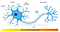In our brains, impulses are received from the Dendrites, get processed in the Nucleus, then get output to the Axon terminals via the Axons.

Artificial Neural Networks are designed with a series of inputs, either from the input layer or from neurons in other layers of the network. There is then a calculation that occurs in what is called an Activation Function. The activation functions are “summed” up and fired across to the output, which deliver the the activation signal to the next layer of the network.

## Basics

Suppose we want to predict whether a student will enter university or not based on her grades and test scores.

The goal of the algorithm is to find a boundary line that keeps most of the blue points above it, and most red points below it.

We might define this by plotting a line, 2x₁ + x₂ -18 = 0

If the student’s score is greater than 0, they are accepted. If it’s less than 0, they are rejected.

This simple strategy would work, but now suppose that instead of just grades and test scores, we want to make a similar prediction while also taking into consideration the student’s class rank. The above model wouldn’t be suitable. We would need to add a third feature to our data set, which would result in having a three-dimensional boundary. This means we’d have three axes, x₁ for the test, x₂ for the grades and x₃ for the class rank.

We’d now calculate w₁x₁ + w₂x₂ + w₃x₃ + b = 0 (weight of the test score, weight of the grades and weight of the class rank, plus some bias). This equation can be simplified to Wx + b = 0

Our prediction ŷ (pronounced “y-hat”) will now tell us if the student is above this division plane (accepted) or below it (rejected) given the three features.

One interesting aspect to point out here is that even if we wanted to add a fourth feature or dimension, such as the student’s SAT score, the equation would remain identical. Our prediction ŷ will still tell us if the student is above this division plane or below it.

So, how might we execute this in a Neural Network?

## Perceptrons

Perceptrons are the building blocks of neural networks. They are merely the encoding of our equation into a small graph. We fit our data and boundary line inside a node. Then we add small nodes for the inputs, which are tests and grades. The perceptron plots the points provided by the inputs and checks whether the result is a pass or a fail. It then output a result.

## Step Function

Notice how the output is either a Yes (pass) or a No (fail). To come up with this result we use what is called a Step Function. This is great for when we need make a binary decision, but not so great when there’s less certainty.

It turns out there are several other functions that can be applied to the output, which I will discuss shortly.

## Perceptron trick

How do we find the line that separates the red points from the blue points in the best possible way? First we need to plot and label our points. Then we draw a line and check how badly it performs the classification of the given points.

Once we’ve found how well or badly the line has performed, we can either move it towards or away from our point. We can use what’s called a Learning Rate, and set it to a very small value so that the line doesn’t move too drastically in one direction or another. We then multiply our original numbers by the learning rate and receive a new equation, which will shift the line. That is the trick we will use repeatedly for the perceptron algorithm.

Say we’re on a mountain-top and want to descend in the fastest way possible. In an ideal world, we would look around us and pick the path that allows us to descend most rapidly in one direction. We’d then repeat the process over and over until we reach our goal. This is called gradient descent.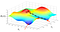If we were on a mountain, we’d descend it by looking around us and finding the place to walk that would most rapidly bring us to the bottom.

In one sentence, Gradient Descent is an iterative algorithm that allows us to move our parameters (or coefficients) towards the optimum values.

## Log-loss Error

Log-loss measures the accuracy of a classifier. We can use such a method to detect the error in our model and then gradually shift the line in one direction or the other via Gradient Descent. We can also assign larger weights to the points that are misclassified so to converge more rapidly (similar to how we’d pick a steeper direction to step towards on a mountain in order to descend quicker.) This will allow the line to move more rapidly towards points that are misclassified. The goal is to ensure the sum of our errors is as small as possible.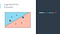We want to sum of our errors to be as small as possible. Image Credits: Udacity — Deep Learning Nanodegree

If two points were merely telling us “I’m not in the right place!” how would we know who to give more importance to? Given the iterative nature of Gradient Descent, rather than the points just telling us if they are properly or improperly classified, it would be far better if they told us with how much confidence they are in the right zone. In other words, it be better if they told us the probability of their correctness. The probability is a function of the point’s distance from the line. With that information, we could calculate the correctness of our model with something called cross-entropy (which I won’t get into much detail here). Suffice it to say that cross-entropy is the sum of the negative logarithms of the probabilities of the points being the right color.

If the error of our function is given by E, then the gradient (∇) of E is given by the vector sum of the partial derivatives of E with respect to w₁ and w₂. Simply put, derivatives are nothing more than a way to show rate of change at a given point. In our case, you can think of them as the slope. The gradient tells us the direction we want to move if we want to increase our error function the most. So if we take the negative of the gradient, this will tell us how to decrease the error function the most.

Simply put: the change we are to make to descend the mountain is given by the combination of a direction and its error.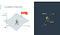We take the negative of the gradient to decrease our error function. Image Credits: Udacity — Deep Learning Nanodegree

Notice how the output we were trying to get earlier was either a Yes or a No (pass or fail). To come up with this result we used what is called a Step Function. If instead of a Step Function, we used a Sigmoid Function, then we will get numbers close to 1 for large positive numbers, and numbers close to 0 for large negative numbers. Isn’t this starting to look a lot like a probability?

Our new perceptron takes the inputs, multiplies them by the weights in the edges, then adds the results. It then applies the sigmoid function. Whereas our previous step function told us whether a student got accepted or rejected, our sigmoid function will tell us the confidence or probability with which the student gets accepted.

## Non-Linear Regions

Consider what would happen if our data were a bit messier and that we couldn’t simply separate the dots with a straight line. In that case we would need a model that can still properly separate students who pass from those who fail, but the line will need to be more complex to make up for the additional criteria. How would we create such non-linear models?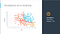If our student got 9/10 on her tests but 1/10 on her school grades, are we sure we want her to pass? This more complicated decision will require a non-linear region to be drawn across our data set. Image Credits: Udacity — Deep Learning Nanodegree

The trick is to combine two linear models into a non-linear model. We then apply the sigmoid function to every point which will give us a curved line.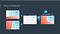By combining two linear regions and applying the sigmoid function, we get a non-linear region. Image Credits: Udacity — Deep Learning Nanodegree

This is precisely what happens in the neural network.

What if we combine a two-node input to a three-node hidden layer?

We will simply get a triangular shaped output layer.

Now, what if we have 3 input nodes? That simply means our output will be a three-dimensional layer. Generally speaking, if we have n-nodes as an input, our output will be in n-dimensional space.

If our output layer has more nodes, then we will get a multi-class classification model.

Finally, what if we have more hidden layers? In that case, we have what’s called a Deep Neural Network.

Our linear models combine to create non-linear models, and in turn, those combine to create even more non-linear models. Lots of hidden nodes can autonomously create highly complex models while not being explicitly programmed to do so. This is precisely where the magic of Neural Networks happens.

## Feedforward

The process of taking inputs, combining their weights to obtain a non-linear model, then combining those to produce a non-linear output is called Feedforward.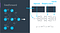Starting from the right, we take a series of inputs, do matrix multiplication over the weights, apply a sigmoid function, then move on to the next later and do the same. The final output is our prediction. Image Credits: Udacity — Deep Learning Nanodegree

## Backpropagation

Once we’ve done a Feedforward operation, we first compare the output of the model with the desired output. We then calculate the error. Once we have that, we run the Feedforward operation backwards (Backpropagation) to spread the error to each of the weights. Then we use this to update the weights and get a better model. We repeat this process until we are happy with the model.

Why does this work? Because while the Feedforward step was telling us the direction and amount that a perceptron should change next time, the Backpropagation step is saying, “if you want that perceptron to be x amount higher, then I am going to have to change these previous perceptrons to be y amount higher/lower because their weights were amplyfying the final prediction by n times”.

In general, feed forwarding is just composing a bunch of functions, and Backpropagation is taking the derivative at each piece to update our weights.

## In conclusion

These are the very basics of Deep Learning and Artificial Neural Networks. The forward and backward flow of calculations that repeatedly adjust themselves have tremendous potential to discover patterns in data.

Many techniques used by Deep Learning have been around for decades, such as the algorithms to recognize hand-written postal codes in the ‘90s. The use of Deep Learning has surged over the past five years due to 3 factors:

1. Deep Learning methods have obtained a higher accuracy than people in classifying images.
2. Modern GPUs allow us to train complex networks in less time than ever before.
3. Massive amounts of data required for Deep Learning has become increasingly accessible.

The basic ANN described above is just the start. There are many more complex models that have been developed in recent years including:

## Convolutional Neural Networks

A class of Deep Neural Networks, most commonly applied to analyzing visual imagery.

## Recurrent Neural Networks

A class of Deep Neural Networks that allows exhibiting temporal dynamic behavior. Unlike standard Feedforward neural networks, RNNs can use their internal state (memory) to process sequences of inputs. This technique is especially good for audio (eg. Apple’s Siri or Amazon’s Alexa).

A class of Deep Neural Networks where multiple neural networks contest with each other in a zero-sum game framework. This technique can generate text, photographs or other inputs that look at least superficially authentic to human observers.

Are Neural Networks the best possible structures for finding patterns in a given data-set? Who knows — it might very well be that in a decade, we will make use of new algorithms that stray significantly from ANNs. That said, I think we’re onto something here.

The baffling aspect of Neural Networks is how well they actually perform in practice. As PhD student at Oxford University and research scientist at DeepMind, Andrew Trask puts it, the extraordinary thing about Deep Learning is that unlike the other revolutions in human history,

this field is more of a mental innovation than a mechanical one. […] Deep Learning seeks to automate intelligence bit by bit.”

Written by

## Daniele Pestilli

#### Full-stack developer, East Asia enthusiast, Artist

Welcome to a place where words matter. On Medium, smart voices and original ideas take center stage - with no ads in sight. Watch
Follow all the topics you care about, and we’ll deliver the best stories for you to your homepage and inbox. Explore
Get unlimited access to the best stories on Medium — and support writers while you’re at it. Just \$5/month. Upgrade